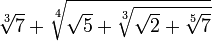# An Algebraic Number$\sqrt{7}+\sqrt{\sqrt{5}+\sqrt{\sqrt{2}+\sqrt{7}}}$